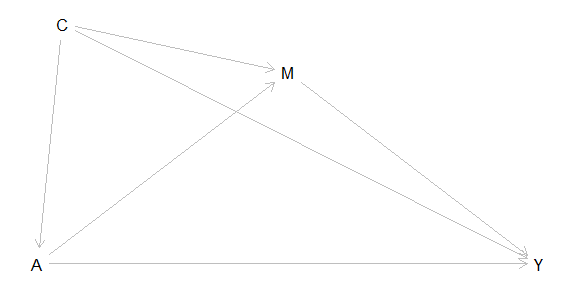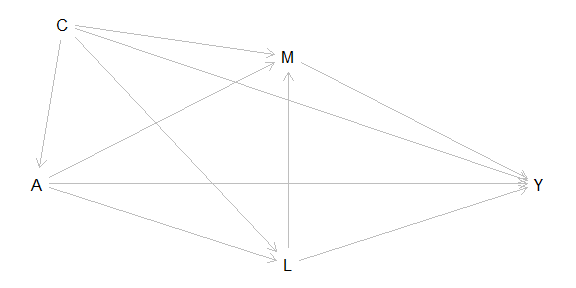The R package CMAverse provides a suite of functions for reproducible causal mediation analysis including cmdag for DAG visualization, cmest for statistical modeling and cmsens for sensitivity analysis.

See the package website for a quickstart guide, an overview of statistical modeling approaches and examples.

### DAG Visualization

cmdag visualizes causal relationships via a directed acyclic graph (DAG).

### Statistical Modeling

cmest implements six causal mediation analysis approaches including the regression-based approach by Valeri et al. (2013) and VanderWeele et al. (2014), the weighting-based approach by VanderWeele et al. (2014), the inverse odd-ratio weighting approach by Tchetgen Tchetgen (2013), the natural effect model by Vansteelandt et al. (2012), the marginal structural model by VanderWeele et al. (2017), and the g-formula approach by Robins (1986).

cmest currently supports a single exposure, multiple sequential mediators and a single outcome. When multiple mediators are of interest, cmest estimates the joint mediated effect through the set of mediators. cmest also allows for time varying confounders preceding mediators. The two causal scenarios supported are:

1. There are no confounders affected by the exposure:1. There are mediator-outcome confounders affected by the exposure and these confounders precede all of the mediators:Table: Supported Data Types and Functionalities of cmest
rb wb iorw ne msm gformula1
Continuous Y2
Binary Y
Count Y
Nominal Y ×
Ordinal Y ×
Survival Y × ×
Continuous M ×
Binary M
Nominal M
Ordinal M
Count M ×
M of Any Type × × ×
Continuous A ×3 × ×4
Binary A
Nominal A
Ordinal A
Count A ×5 × ×6
Mediator-outcome Confounder(s) Affected by A × × × ×
2-way Decomposition
4-way Decomposition ×
Estimation: Closed-form Parameter Function 7 × × × × ×
Estimation: Direct Counterfactual Imputation
Inference: Delta Method 8 × × × × ×
Inference: Bootstrapping
Marginal Effects 9
Effects Conditional on C 10 × × × × ×
1. rb: the regression-based approach; wb: the weighting-based approach; iorw: the inverse odds ratio weighting approach; ne: the natural effect model; msm: the marginal structural model; gformula: the g-formula approach.↩︎

2. Y denotes the outcome, A denotes the exposure, M denotes the mediator(s) and C denotes the exposure-outcome confounder(s), the exposure-mediator confounder(s) and the mediator-outcome confounder(s) not affected by the exposure.↩︎

3. continuous A is not supported when C is not empty; otherwise, it is supported.↩︎

4. continuous A is not supported when C is not empty; otherwise, it is supported.↩︎

5. count A is not supported when C is not empty; otherwise, it is supported.↩︎

6. count A is not supported when C is not empty; otherwise, it is supported.↩︎

7. closed-form parameter function estimation only supports a single mediator.↩︎

8. delta method inference is available only when closed-form parameter function estimation is used.↩︎

9. marginal effects are estimated when direct counterfactual imputation estimation is used.↩︎

10. conditional effects are estimated when closed-form parameter function estimation is used.↩︎

### Multiple Imputation

cmest provides the option multimp = TRUE to perform multiple imputations for a dataset with missing values.

### Sensitivity Analysis

cmsens conducts sensitivity analysis for unmeasured confounding via the E-value approach by VanderWeele et al. (2017) and Smith et al. (2019), and sensitivity analysis for measurement error via regression calibration by Carroll et al. (1995) and SIMEX by Cook et al. (1994) and Küchenhoff et al. (2006). Sensitivity analysis for measurement error is currently available for the regression-based approach and the g-formula approach.

## Installation

devtools::install_github("BS1125/CMAverse")
Load CMAverse:
library(CMAverse)